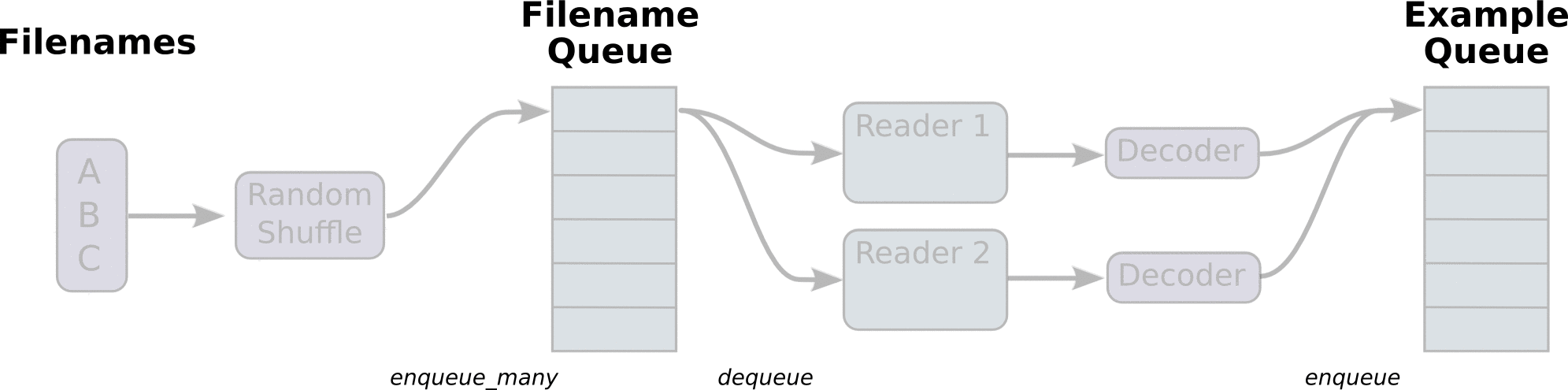Jarvis' Blog (总有美丽的风景让人流连) 总有美丽的风景让人流连

# Tensorflow 数据输入管线

2018-04-28
Jarvis
Post## 1. 数据预处理: Data —> TFRecord

### 1.1 数据协议栈的简略分析

1.1 节介绍一下 TF 中序列化数据的表达方式, 不感兴趣可以跳过, 直接看 1.2 节.

``````1
2
3
message Example {
Features features = 1;
};
``````

``````1
2
3
4
message Features {
// Map from feature name to feature.
map<string, Feature> feature = 1;
};
``````

``````1
2
3
4
5
6
7
8
message Feature {
// Each feature can be exactly one kind.
oneof kind {
BytesList bytes_list = 1;
FloatList float_list = 2;
Int64List int64_list = 3;
}
};
``````

``````1
2
3
4
5
6
7
8
9
message BytesList {
repeated bytes value = 1;
}
message FloatList {
repeated float value = 1 [packed = true];
}
message Int64List {
repeated int64 value = 1 [packed = true];
}
``````

• 只有原始数值类型的重复字段才可以声明为 `packed`
• 使用 `packed` 后表示该字段会采用更加紧凑的编码格式, 同时不会造成数据的损失.

### 1.2 创建 `Example` 对象

``````1
2
3
4
5
6
7
8
9
10
11
12
13
14
15
16
17
18
19
20
21
# 创建要写入的文件
record_writer = tf.python_io.TFRecordWriter(filename)
# 图像数组, 标签, 图像大小, 图像名称
image = np.arange(3*1024*768).reshape(3, 1024, 768)
label = 1
height, width = 1024, 768
name = "a_string"
# 定义 Example 对象
example = tf.train.Example(
features = tf.train.Features(
feature = {
"i": tf.train.Feature(bytes_list=tf.train.BytesList(value=[image.tobytes()])),
"n": tf.train.Feature(bytes_list=tf.train.BytesList(value=[str.encode(name)])),
"l": tf.train.Feature(int64_list=tf.train.Int64List(value=[label])),
"h": tf.train.Feature(int64_list=tf.train.Int64List(value=[height])),
"w": tf.train.Feature(int64_list=tf.train.Int64List(value=[width]))
}
)
)
# 写入文件
record_writer.write(example.SerializeToString())
``````

## 2. 创建数据输入管线 Data —> Input

• 最简单的方法, 完全在 python 中准备数据, 使用 `tf.placeholder` 作为数据占位符, 训练/验证的每一步送入一批新的数据
• 消耗 NumPy 数组. 当所有的数据都已经载入内存时, 可以直接从数组创建数据集(警告: 此种方法会多次复制数组内容, 慎用)
• 消耗 TFRecord 数据. 当使用大型数据集(如图像数据集)时, 最好用这种方法.
• 消耗文本数据. 略

### 2.1 使用 Python 准备数据

``````1
2
3
4
5
6
7
8
9
10
image = tf.placeholder(tf.float32, shape=[batch_size, None, None, channels])
label = tf.placeholder(tf.float32, shape=[batch_size])

# 直接使用 image 和 label 构建计算图
...

# 在 Python 中创建数据生成器, 每次迭代中产生一批数据并执行计算图
for i in range(max_iter):
image_batch, label_batch = data_generator.next()
sess.run(fetches, feed_dict={image: image_batch, labels: label_batch})
``````

### 2.2 从 NumPy 数组创建数据集

``````1
2
# features: [num_examples, ...], labels: [num_examples]
dataset = tf.data.Dataset.from_tensor_slices((features, labels))
``````

``````1
``````

### 2.2 从 TFRecord 创建 TF 数据集

``````1
2
3
4
# 数据集只能便利一次
dataset = tf.data.TFRecordDataset(filenames)
# 数据集无限遍历
dataset = tf.data.TFRecordDataset(filenames).repeat()
``````

``````1
dataset = dataset.prefetch(2 * batch_size)
``````

``````1
dataset = dataset.map(parser, num_parallel_calls=2)
``````

``````1
2
min_queue_samples = num_examples_per_epoch * 0.4 + 3 * batch_size
dataset = dataset.shuffle(buffer_size=min_queue_samples)
``````

``````1
2
3
4
dataset = dataset.batch(batch_size)
# 创建迭代器, 由于我们一开始已经对数据集设置了 repeat 属性, 所以 one shot 的迭代器仍然可以无限迭代
iterator = dataset.make_one_shot_iterator()
image_batch, label_batch = iterator.get_next()
``````

``````1
2
3
4
5
6
7
8
image_batch, label_batch = tf.data.TFRecordDataset(filenames) \
.repeat() \
.prefetch(2 * batch_size) \
.map(parser, num_parallel_calls=2) \
.shuffle(buffer_size=min_queue_samples) \
.batch(batch_size) \
.make_one_shot_iterator() \
.get_next()
``````

#### 映射函数

``````1
2
3
4
5
6
7
8
9
10
11
12
13
14
15
16
17
18
19
20
21
def parser(serialized_example):
# 这里解析数据所使用的 features 的格式必须与创建 TFRecord 时一致.
features = tf.parse_single_example(
serialized_example,
features={
"image": tf.FixedLenFeature([], tf.string)
"label": tf.FixedLenFeature([], tf.int64)
}
)
# 这里的类型应当与保存图像时的类型一致
image = tf.decode_raw(features["image"], tf.float32)
image.set_shape([DEPTH * HEIGHT * WIDTH])
label = tf.cast(label, tf.int32)

# 调整通道
image = tf.transpose(tf.reshape(image, [DEPTH, HEIGHT, WIDTH]), [1, 2, 0])

# 数据增广
image = data_augmentation(image)

return image, label
``````

#### 使用同一个数据集对象产生不同的数据集迭代器

``````1
2
3
4
5
6
7
8
9
10
11
# 创建文件名的占位符
filenames = tf.placeholder(tf.string, shape=[None])
dataset = tf.data.TFRecordDataset(filenames)
dataset = ...
iterator = dataset.make_initializable_iterator()
# 使用训练数据集初始化数据集对象
training_filenames = ["trainset1.tfrecord", "trainset2.tfrecord"]
sess.run(iterator.initializer, feed_dict={filenames: training_filenames})
# 使用验证数据集初始化数据集对象
validation_filenames = ["validation1.tfrecord", ...]
sess.run(iterator.initializer, feed_dict={filenames: validation_filenames})
``````

Content